Experiment No: 08

Title : WAVEFORM GENERATOR

AIM: To design and test square and triangular waveform generator using IC 741

APPARATUS: Experimental Chasis, Resistors, Connecting wires, DMM, LM 741,

Dual power supply, CRO.

CIRCUIT DIAGRAM: Square and Triangular Wave Generator: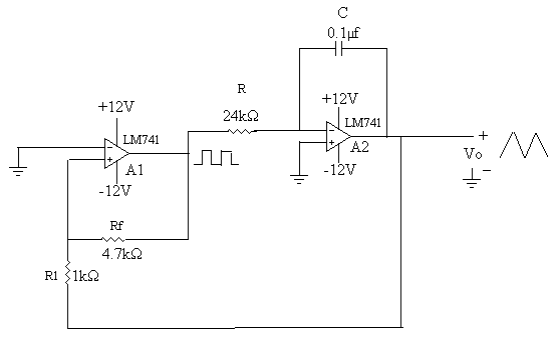THEORY : The circuit consists of a two operational amplifiers. The first amplifier is

non-inverting type of Schmitt trigger i.e. positive feedback. The second amplifier

is an ideal inverting integrator. The output of amplifier A1 is a square wave with

amplitude of Â± Vsat. This output is applied to the inverting integrator, which

produces a triangular wave at the output. To complete the feedback path output of

integrator (i.e. triangular wave) is applied as input to the Schmitt trigger.

Initially let us assume that the output of amplifier A1 is at +Vsat. This is

acting as input to amplifier A2, so its output is negative going ramp. Thus one end

of voltage divider Rf -R1 is at +Vsat and other end is at negative going ramp of A2.

When negative going ramp attains a value of â€“VT, output of A1 switches from

+Vsat to â€“Vsat. The output of A2 now increases in positive direction. When a

positive going ramp attains a +VT, output of A1 switches from â€“Vsat to +Vsat.

This cycle repeats to generate square & triangular waveforms.

The frequencies of both square and triangular waves are same but their

amplitudes are different. The amplitude of square wave is a function of supply

voltages. The desired amplitude can be obtained by connecting zener diodes at

Integrated Circuits & Applications (ICA) Dept. of E&TC Engg.,JSPMâ€™s ICOER, Pune the output of amplifier A1. Amplitude of triangular wave depends on R and C

values of an integrator and also output of amplifier A1.

Design Equations:

For Schmitt Trigger,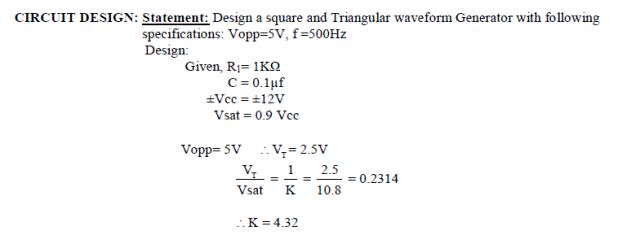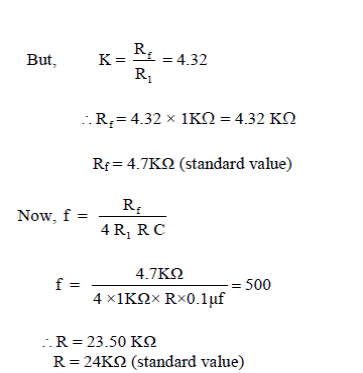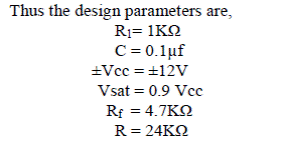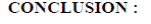------------------------------------------------------------------------------------------------------------------------------------------------------------------------------------------------------------------------------------------------------------------------------------------------------------------------------------------------------------------